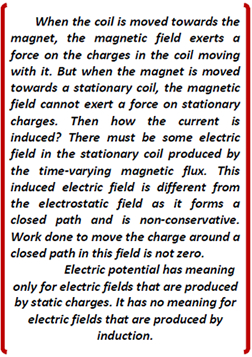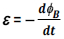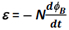On the basis of his experiments (discussed above), Faraday concluded that an emf is induced in a coil when magnetic flux through the coil changes with time. Due to the relative motion between a magnet and a coil X (figure 1) or between a current-carrying coil and a coil X (figure 2) or due to a change in current in one coil (figure 3), the magnetic flux associated with coil X (figure 1 and 2) and coil S (figure 3) changes. The change in magnetic flux induces emf in coil X or coil S. This induced emf set up electric current to flow in coil X or coil S and the galvanometer connected in these coils shows a deflection.

In the setup of experiment 3, current and hence the magnetic flux in the primary coil P changes only during the make or break of the key K and as a result an emf is induced in the secondary coil S. Therefore, the galvanometer shows a momentary deflection only during the make or break of the key K.

Faraday's law of electromagnetic induction was based on his experimental observations.According to this law,

Whenever the magnetic flux through a coil or loop of wire is changed, an emf is induced in the coil or loop. The magnitude of the induced emf is proportional to the rate of change of flux through the coil.

Mathematically, the induced emf is given byThe negative sign indicates the direction of induced emf ε and hence the direction of current in a closed loop. It will be explained on the basis of Lenz's law.

If the loop is replaced by a coil of N turns, then the induced emf is given byIt is clear from this relation that emf may be generated-

1. by changing B with time (experiments 1 and 2),
2. by changing A with time (stretching or shrinking the coil),
3. by changing the orientation of the loop, i.e. by rotating the loop in the field.

CBSE Electromagnetic Induction ( With Hint / Solution)
Class XII (By Mr. Ashis Kumar Satapathy)
email - [email protected]

Electromagnetic Induction